# Intermediate value theorem facts for kids

Kids Encyclopedia Facts

The intermediate value theorem says that if a function,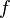$f$, is continuous over a closed interval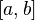$[a,b]$, and is equal to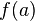$f(a)$ and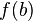$f(b)$ at either end of the interval, for any number, c, between$f(a)$ and$f(b)$, we can find an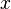$x$ so that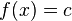$f(x) = c$.

This means that if a continuous function's sign changes in an interval, we can find a root of the function in that interval. For example, if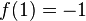$f(1) = -1$ and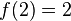$f(2) = 2$, we can find an$x$ in the interval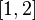$[1,2]$ that is a root of this function, meaning that for this value of x,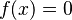$f(x)=0$, if$f$ is continuous. This corollary is called Bolzano's theorem.Intermediate value theorem Facts for Kids. Kiddle Encyclopedia.